Class overview

You learning to teach the computer to learn from data

• How?
• Find a general rule (i.e., 일반화 성능 향상, 오버피팅 방지)
• That explains data given only as a sample of limited size
• According to some measurement of accuracy or error
• 한마디로, finding signals among the noise
• 지금까지 배운 방법론들
• Supervised learning
• Data are sample of input-output pairs
• Find input-output mapping
• Regression, classification, etc
• Unsupervised learning
• Data are sample of objects
• Find some common structure
• Clustering, etc.
• Text mining
• 오늘 배울 것: 여전히 핫한 알고리즘, SVM, and yet another supervised learning algorithm
• 앞으로 남은 세 시간:
• classes[-3]: Semisupervised learning + A touch of visualization
• classes[-2]: Big data technologies: Hadoop & spark + Tips for your exam
• classes[-1]: 대망의 기말고사

SVM: Support vector machines

Classification examples

1. Sheep vector machines
• Using the existing sheep distributions (the training set), determine whether the new sheep belongs with the white sheep or the black sheep.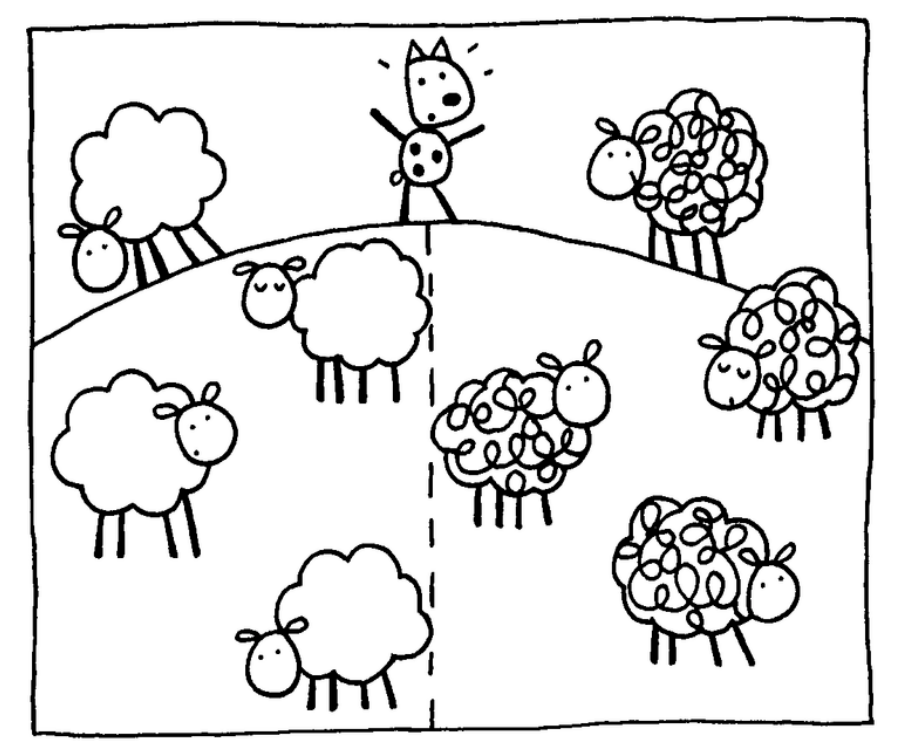2. Spam filtering
• Using word occurrences in existing email documents, determine whether a new email is spam or ham.• Instance space: $x \in X$ ($|X| = n$ data points)
• Binary or real-valued feature vector $x$ of word occurrences
• $d$ features (words + other things, d~100,000+)
• Class: $y \in Y$
• $y$: Spam (+1), Ham (-1)

viagralearningthedatingnigeriais_spam
101001
01100-1
000011

Linear binary classification

• There may exist many solutions that separate the classes exactly
• Usually, we find the one that will give the smallest generalization error
• This is the problem of choosing the or decision boundary, or hyperplane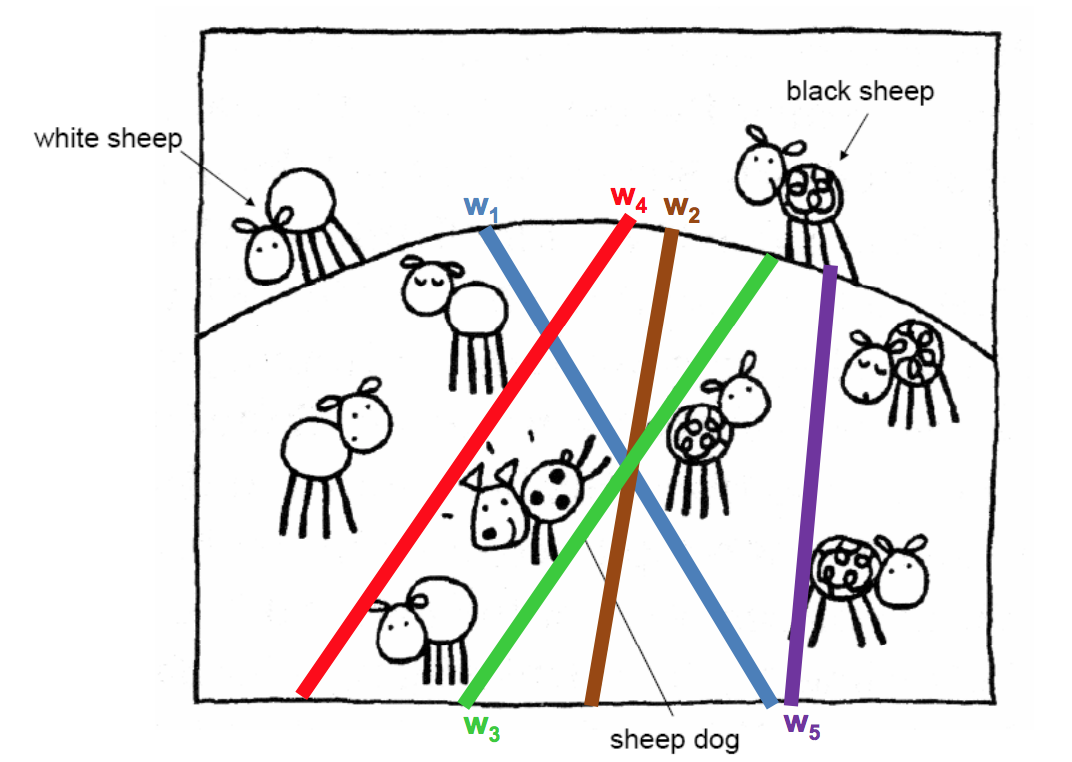• Input: Binary/real valued vectors $x$ and labels $y$
• Goal: Find real valued vector $w$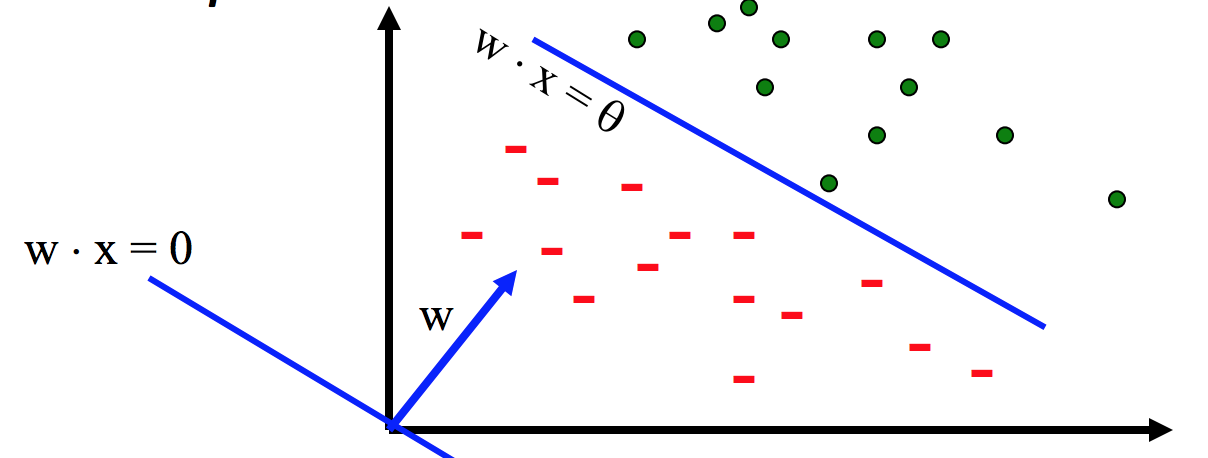• Each feature has a weight $w_i$
• Prediction is based on the weighted sum: $f(x) = \sum w_i x_i = w \cdot x$
• If the f(x) is
• Positive: Predict +1 (i.e., is sheep, is spam)
• Negative: Predict -1 (i.e., is not sheep, is ham)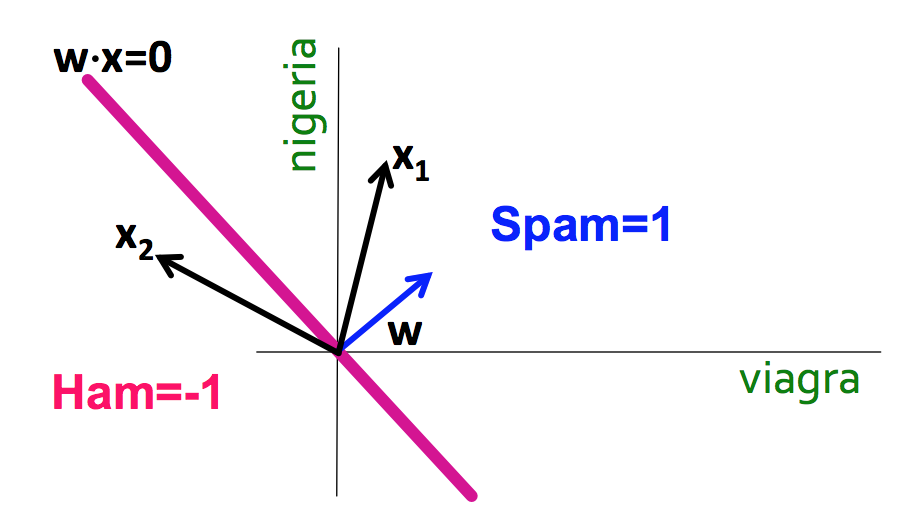SVM, the maximal margin classifier

• Idea:
• Distance from the separating hyperplane corresponds to the "confidence" of prediction
• In the image below, we are more sure about the class of A and B than of C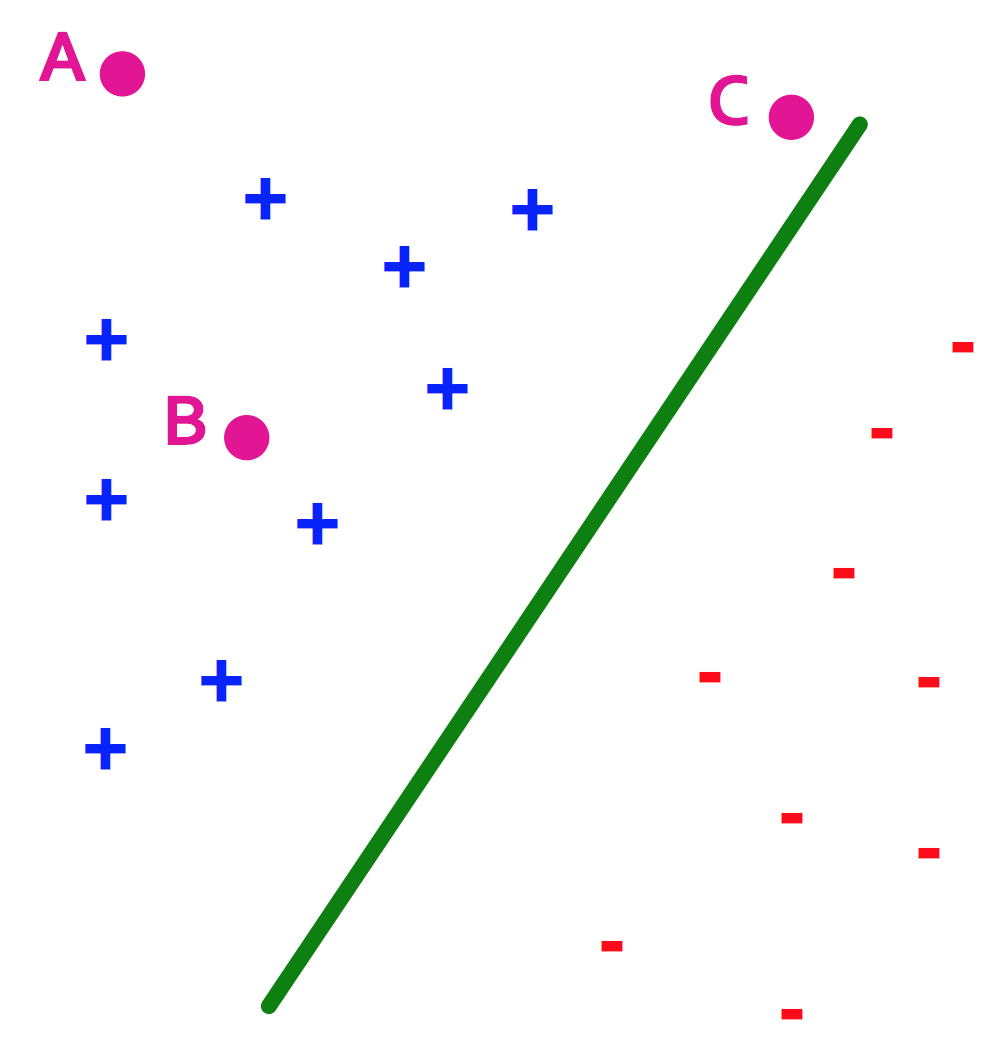• SVM finds the decision boundary with concept of maximizing this distance, or margins
• Margin $\gamma$: The perpendicular distance between the decision boundary and the closest of the data points (left figure below).
• Support vectors: Maximizing the margin leads to a particular subset of existing data points (right figure below).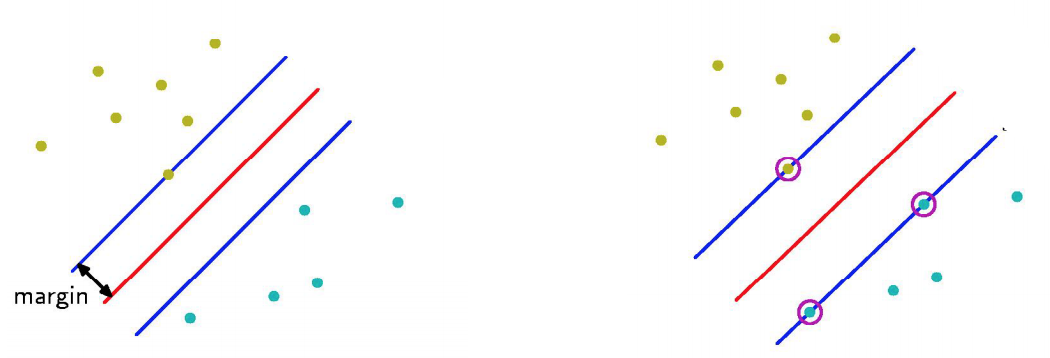• Why is maximizing $\gamma$ a good idea?
• Remember: Dot product $A \cdot B = |A||B|cos\theta$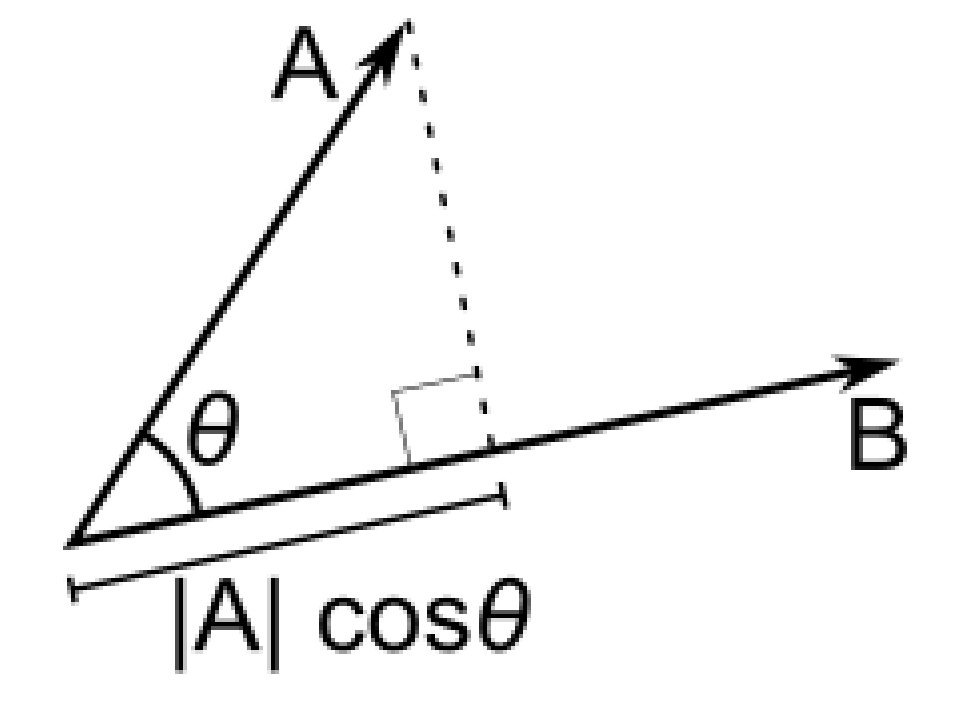• Let:
• Line $L = w \cdot x + b = 0$
• Weight $w = [w_1, w_2]$
• Point $A = [x_1^{(A)}, x_2^{(A)}]$
• Point $M = [x_1^{{(M)}}, x_2^{{(M)}}]$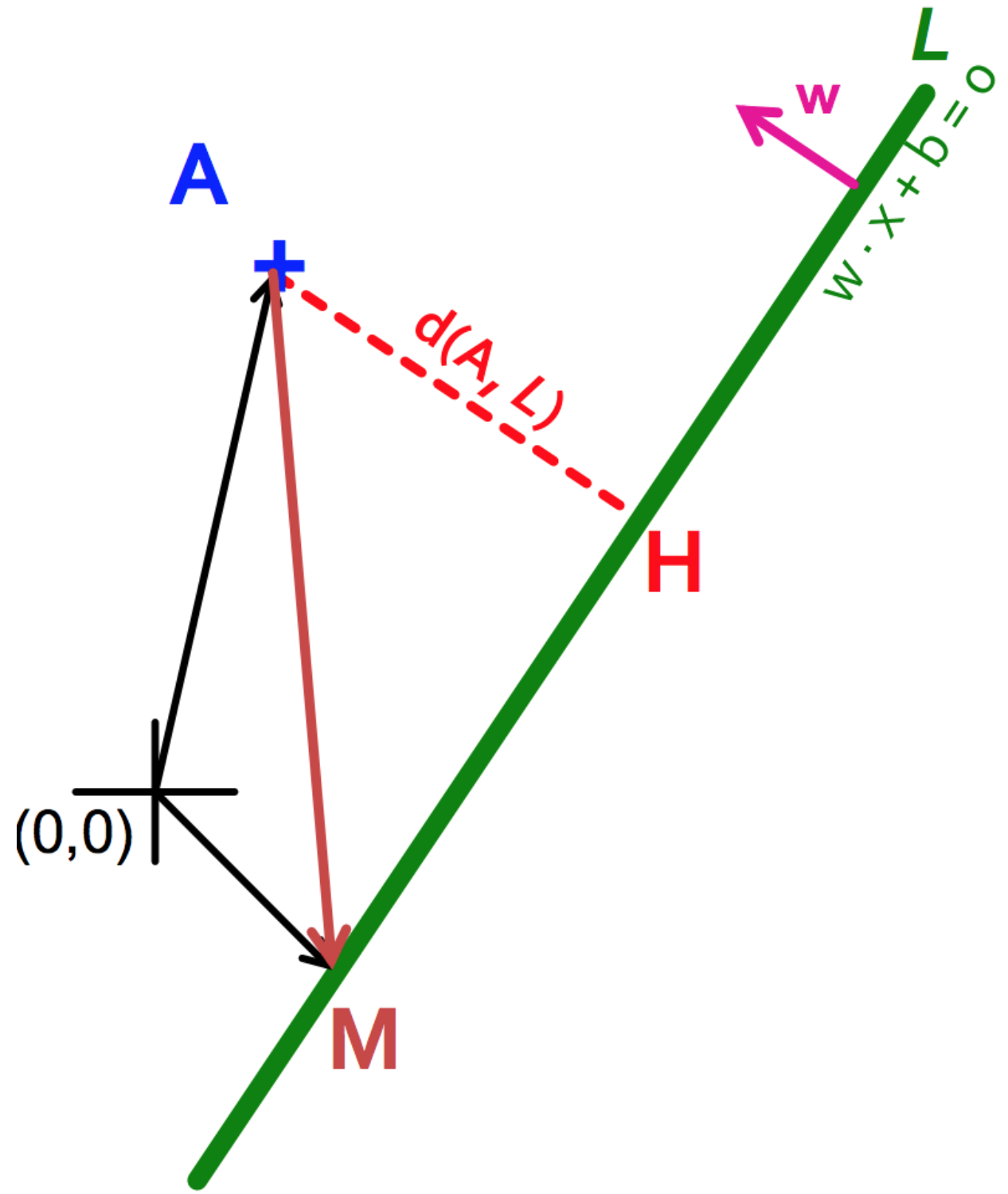• Then the distance between A, L: \begin{align} d(A, L) & = |AH|\\ & = |(A-M) \cdot w|\\ & = |(x_1^{(A)} - x_1^{(M)}) w_1 + (x_2^{(A)} - x_2^{(M)}) w_2|\\ & = x_1^{(A)} w_1 + x_2^{(A)} w_2 + b\\ & = w \cdot A + b \end{align}
• Prediction = $sign(w \cdot x + b)$
• "Confidence" = $(w \cdot x + b)y$
• For i-th data point: $\gamma_i = (w \cdot x^{(i)} + b)y^{(i)}$
• Therefore, the objective function becomes:
• max $\gamma$ s.t., $y^{(i)}(w \cdot x^{(i)} + b) \geq \gamma$ ($\forall i$)
• Good according to 1) intuition 2) theory and 3) practice
• Normalized weights
• Problem: With this equation, scaling $w$ increases margin! (i.e., $w$ can be arbitrarily large)
• If $(w \cdot x + b)y = \gamma$, then $(2w \cdot x + 2b)y = 2\gamma$
• Solution: work with normalized $w$, and require support vectors to be on the margin
• $\gamma = (\frac{w}{|w|} \cdot x + b)y$
• $w \cdot x^{(i)} + b = \pm 1$
• Margin maximization == weight minimization?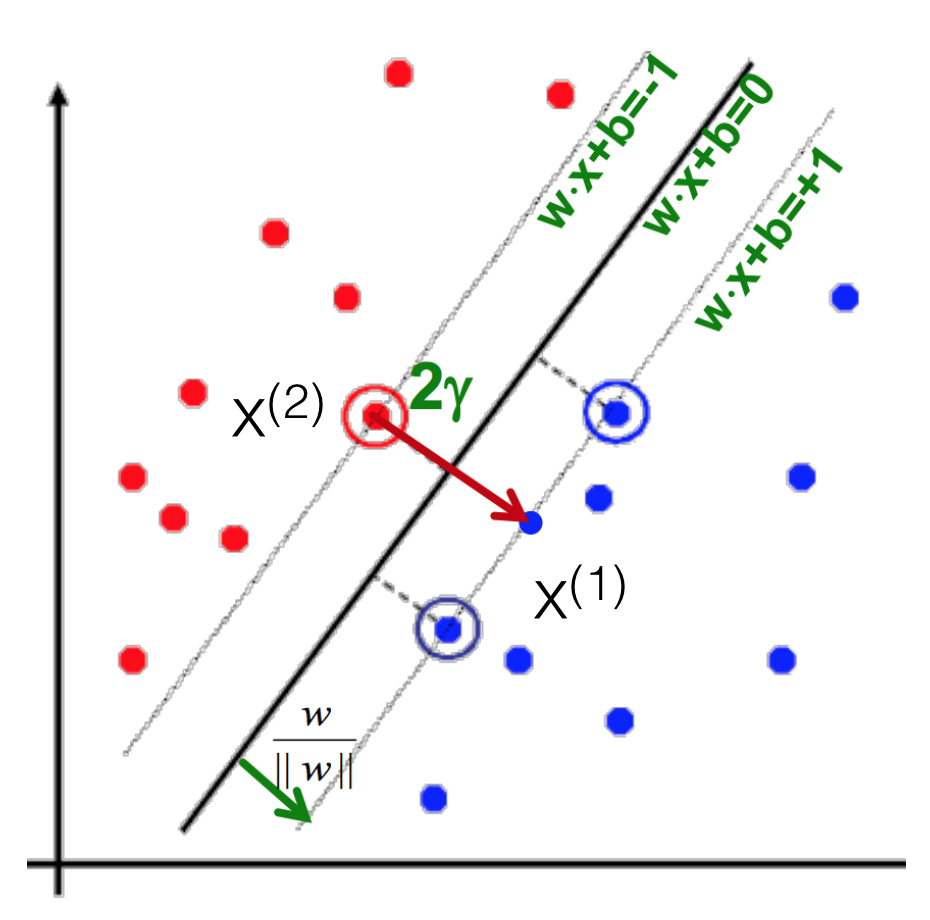• We know three things
• $x^{(1)} = x^{(2)} + 2 \gamma \frac{w}{|w|}$
• $w \cdot x^{(1)} + b = +1$
• $w \cdot x^{(2)} + b = -1$
• Therefore
• $w \cdot x^{(1)} + b = +1$
• $w (x^{(2)} + 2 \gamma \frac{w}{|w|}) + b = +1$
• $(w \cdot x^{(2)} + b) + 2 \gamma \frac{w}{|w|} = +1$
• $\gamma = \frac{1}{|w|}$
• max $\gamma \thickapprox$ max $\frac{1}{|w|} \thickapprox$ min $|w| \thickapprox$ min $\frac{1}{2} |w|^2$
• Which finally gives
• min $\frac{1}{2} |w|^2$ s.t., $y^{(i)}(w \cdot x^{(i)} + b) \geq 1$ ($\forall i$)
• This is called SVM with "hard" constraints

Soft margin SVMs

• Relax constraints
• Allowing the margin constraints to be violated
• In other words, allow some of the training data points to be misclassified
• min $\frac{1}{2} |w|^2 + C \sum_{i=1}^n \xi_i$ s.t., $y^{(i)}(w \cdot x^{(i)} + b) \geq 1 - \xi_i$ ($\forall i$)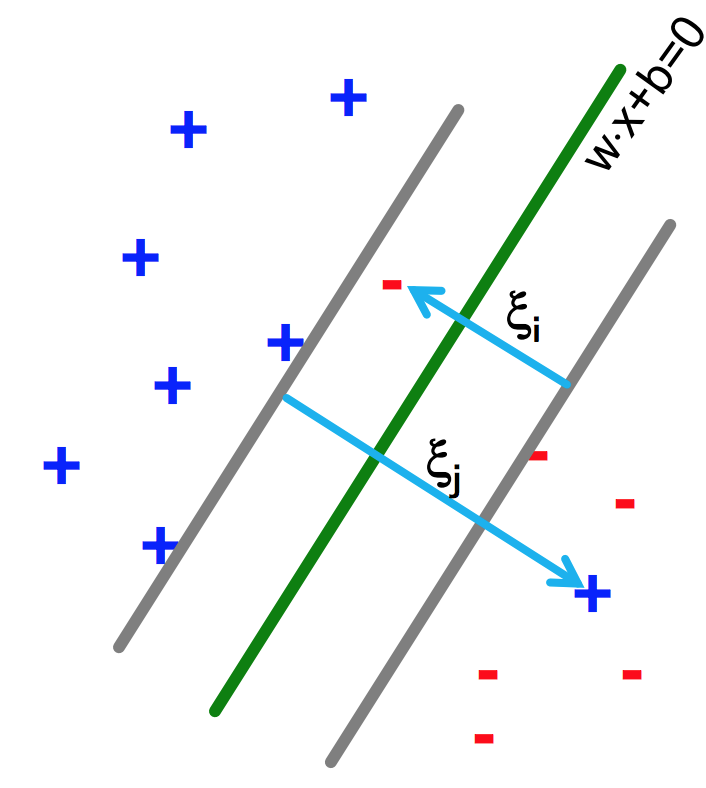Kernel methods

Warning: NOT related to shell/kernels in the OS

• Life is not so easy, not all problems are linearly separable
• If so, choose a mapping to some (high dimensional) dot-product space, namely the feature space: $\Phi: X \to H$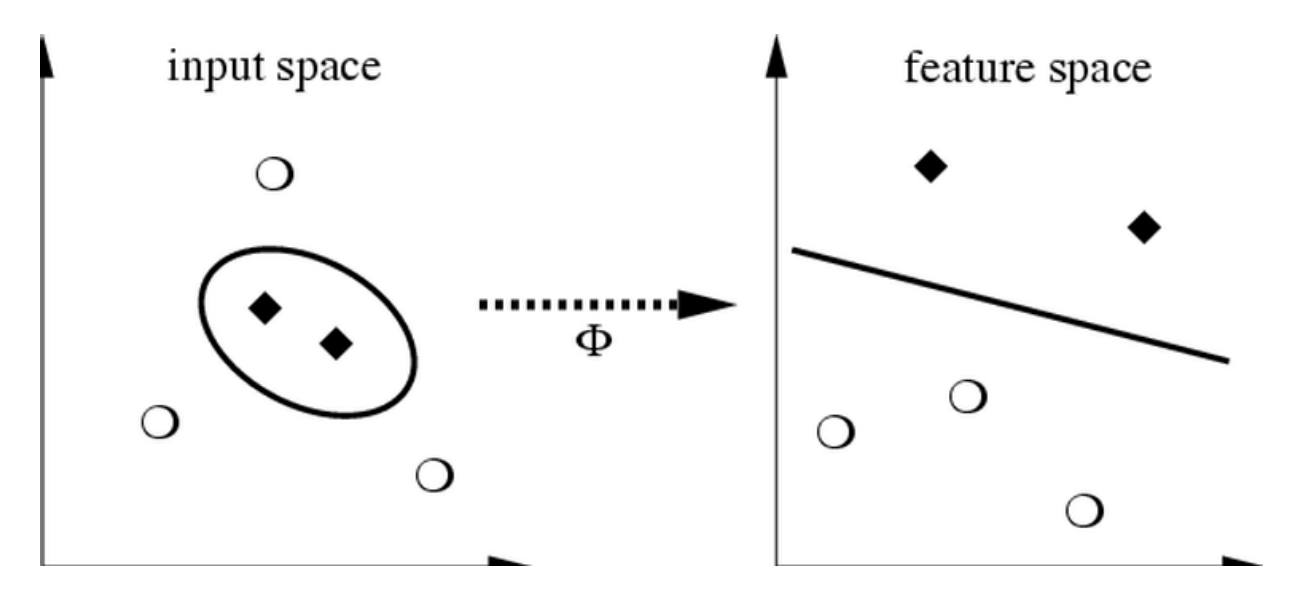• Mercer's condition
• If a symmetric function $K(x, y)$ satisfies $\sum_{i,j=1}^{M} a_ia_jK(x_i,x_j) \geq 0$
for all $M \in \mathbb{N}, x_i, a_i \in \mathbb{R}$
there exists a mapping function $\Phi$ that maps x into the dot-product feature space and $K(x, y) = <\Phi(x), \Phi(y)>$ and vice versa.
• Function $K$ is called the kernel.
• Types of kernels
• Linear kernels: $K(x, y) = <x, y>$
• Polynomial kernels: $K(x, y) = (< x, y> + 1)^d$ for $d = 2$
• RBF kernels: $K(x, y) = exp(-\frac{||x-y||^2}{d^2})$
• ...and more!
• Kernels on various objects, such as graphs, strings, texts, etc.
• Enable us to use dot-product algorithms
• Measure of similarity

Programming SVMs

Go to the SVM documents in the Scikit-learn webpage.

References

Many contents in courtesy of Jure Leskovec and Petra Kudova